• 本文为深度学习神经网络入门文章, 基于Introduction to Deep Learning此篇文章 本文介绍了深度学习中，建立神经网络的三个基本步骤。 第一步：Establish the Structure 建立基本神经结构 第二步： Goodness of ...
本文为深度学习神经网络入门文章, 基于Introduction to Deep Learning此篇文章

本文介绍了深度学习中，建立神经网络的三个基本步骤。
第一步：Establish the Structure 建立基本神经结构
常见基本网络结构： MNIST 256 to 10
第二步： Goodness of Function 准备训练数据以及Loss Function
Loss: Eu Distance, Cross_Entropy，以及各个论文中的新Loss Function
第三步： Optimization 进行优化，使用梯度下降，反向传播法，减少Loss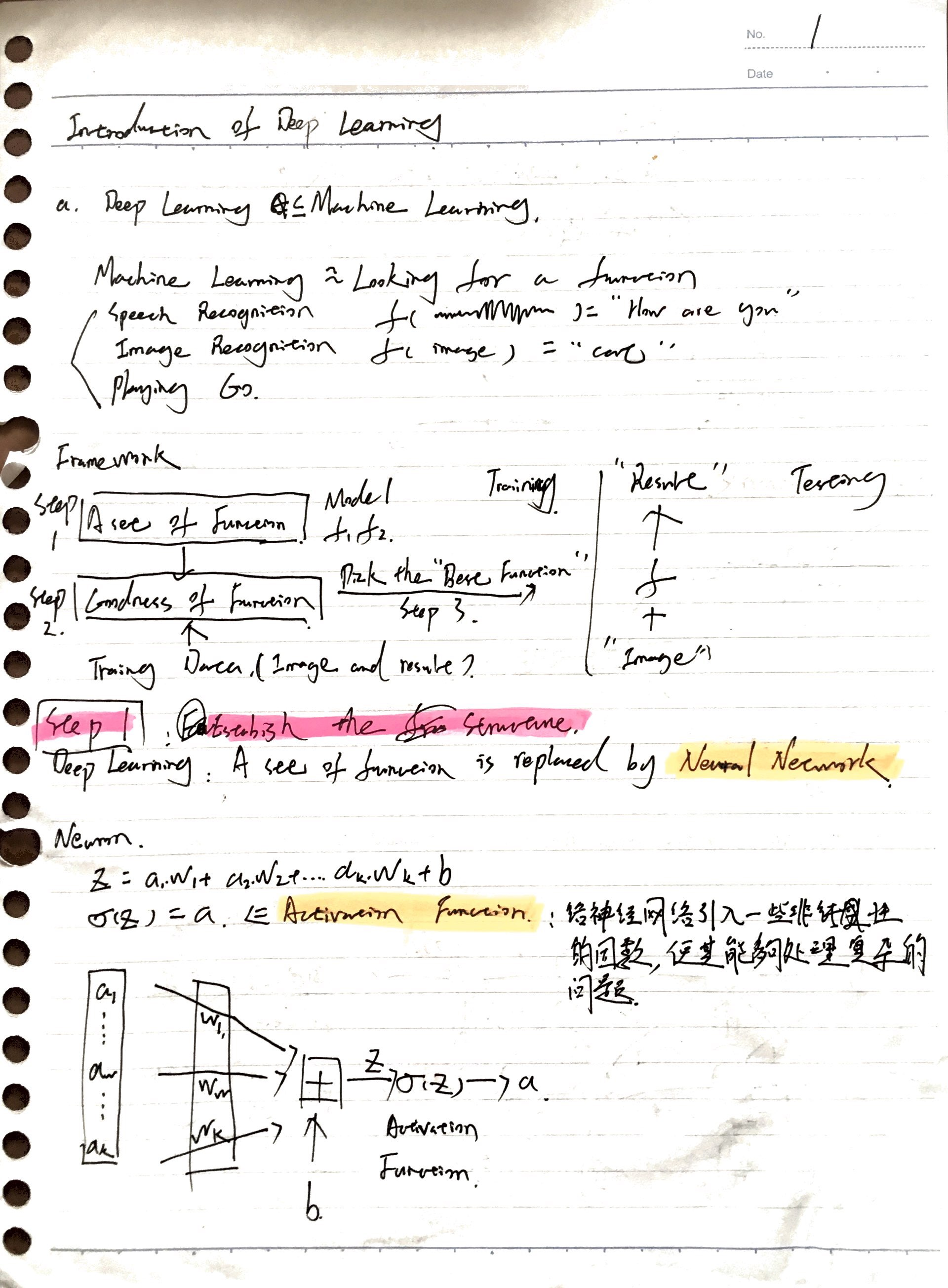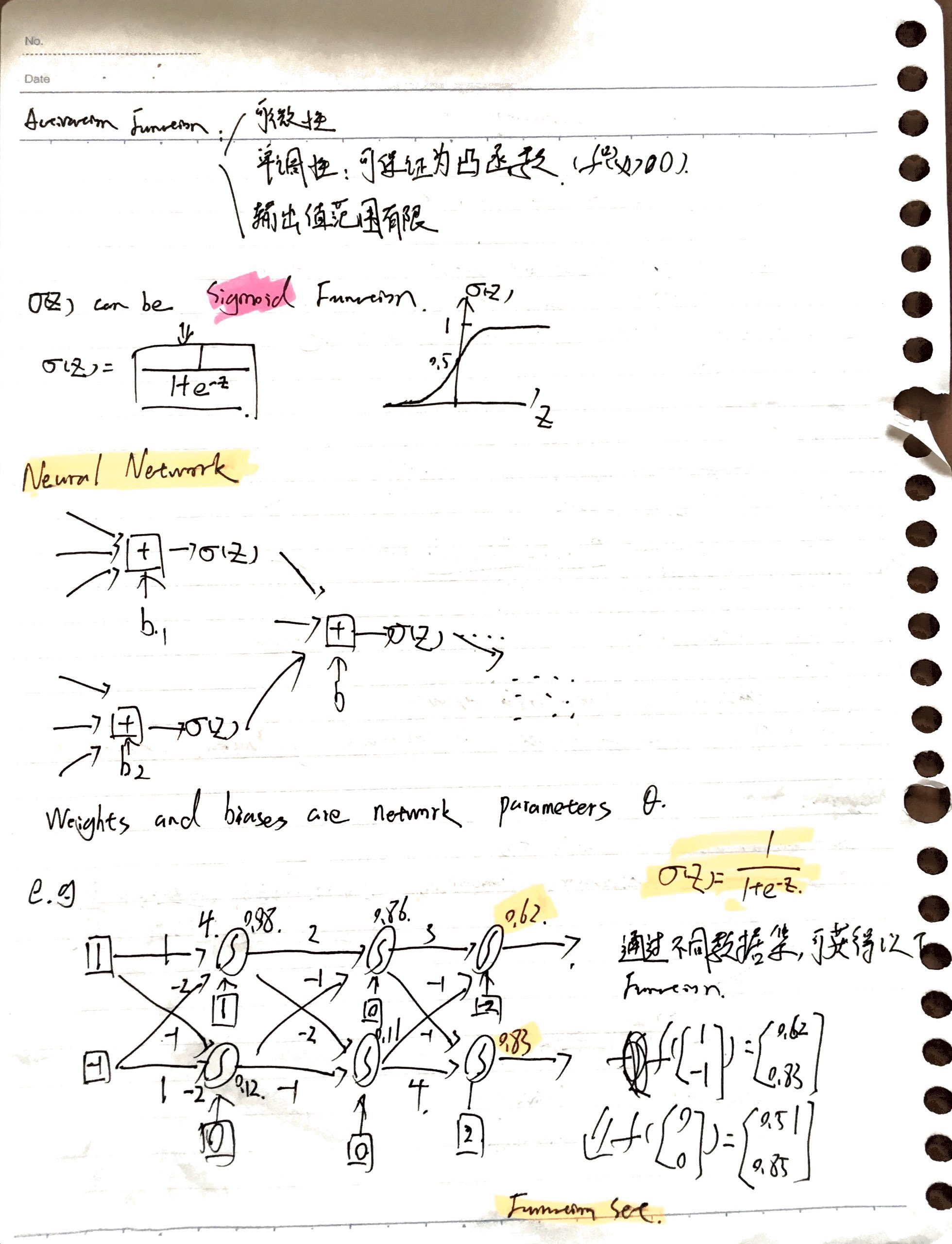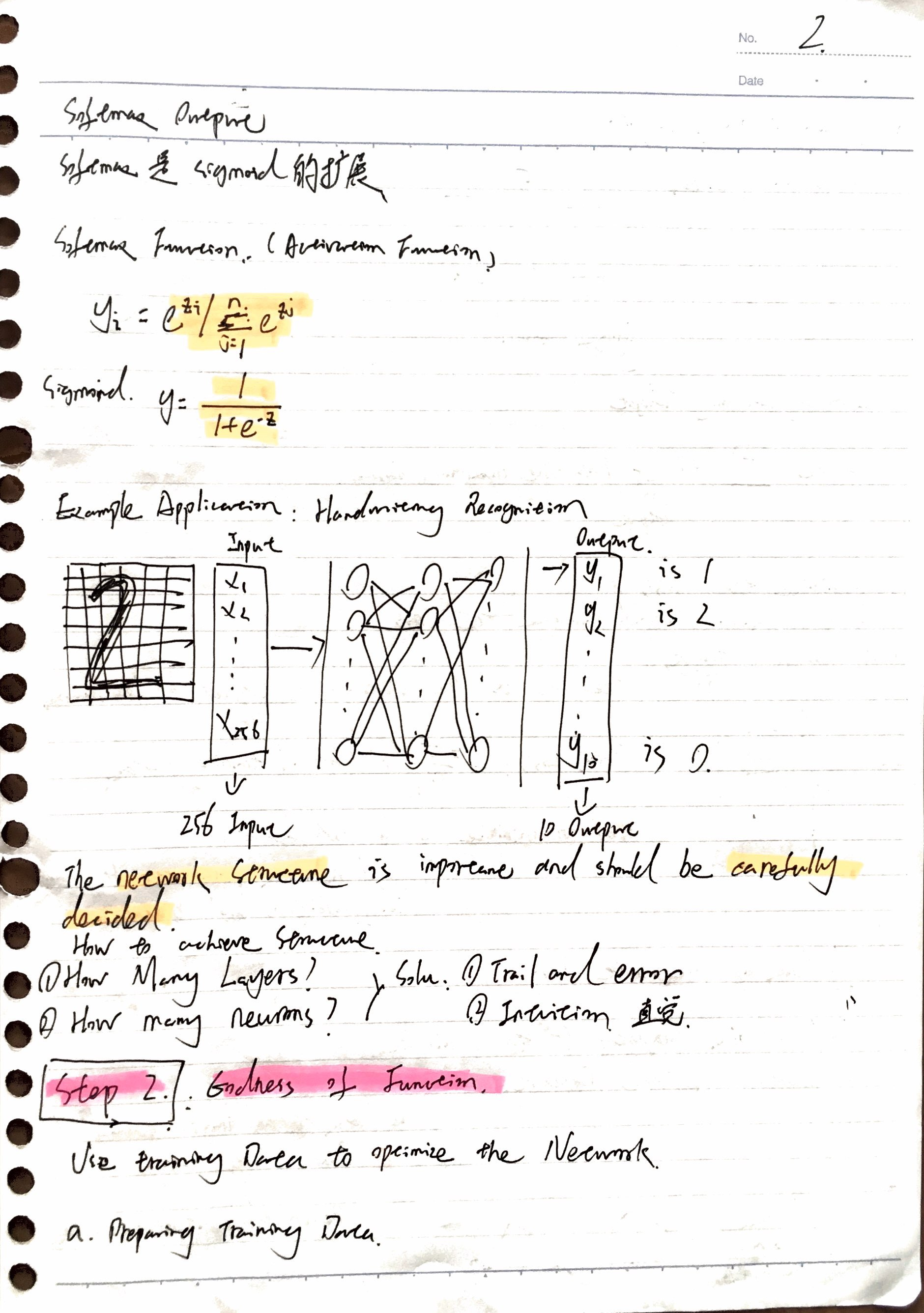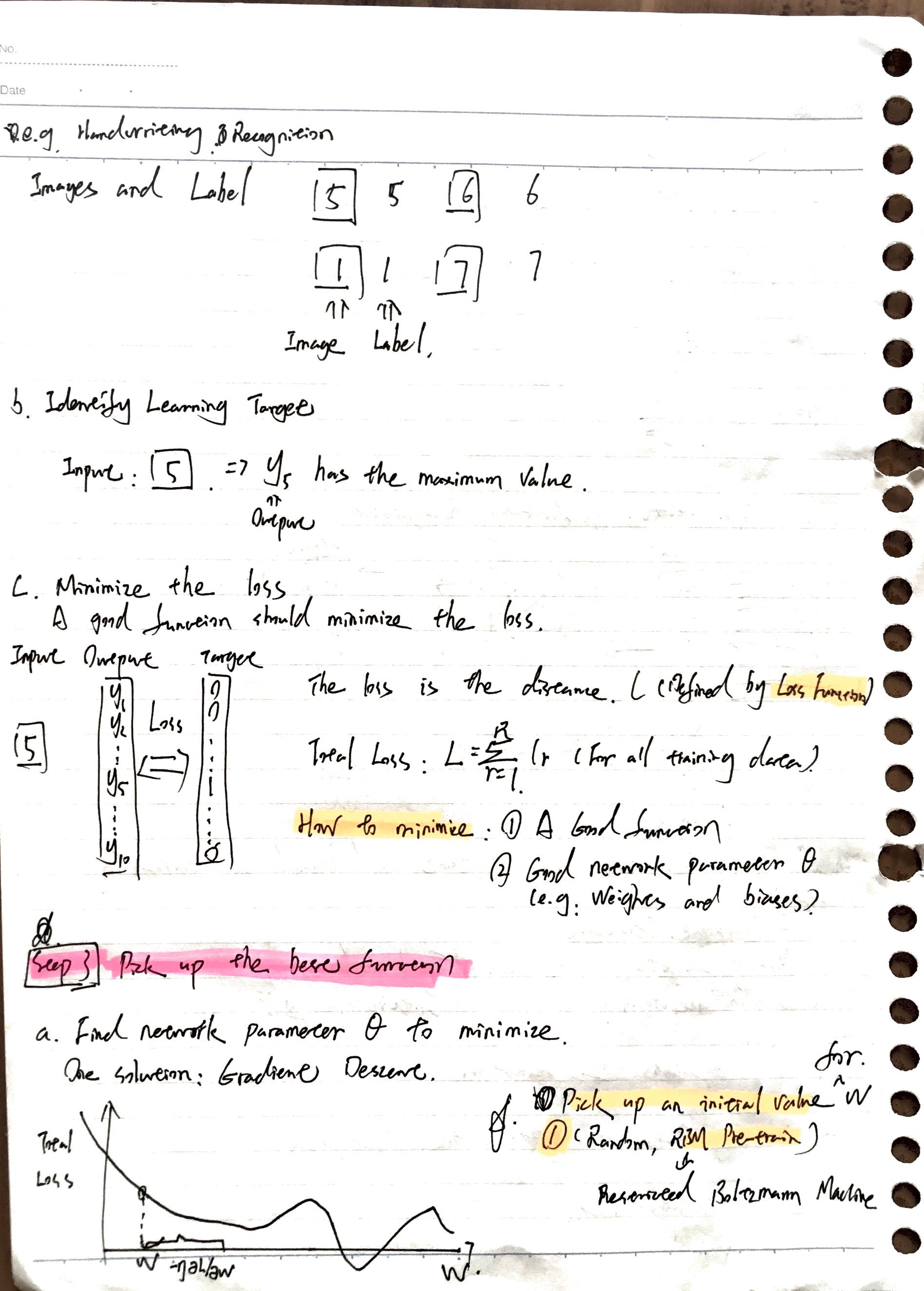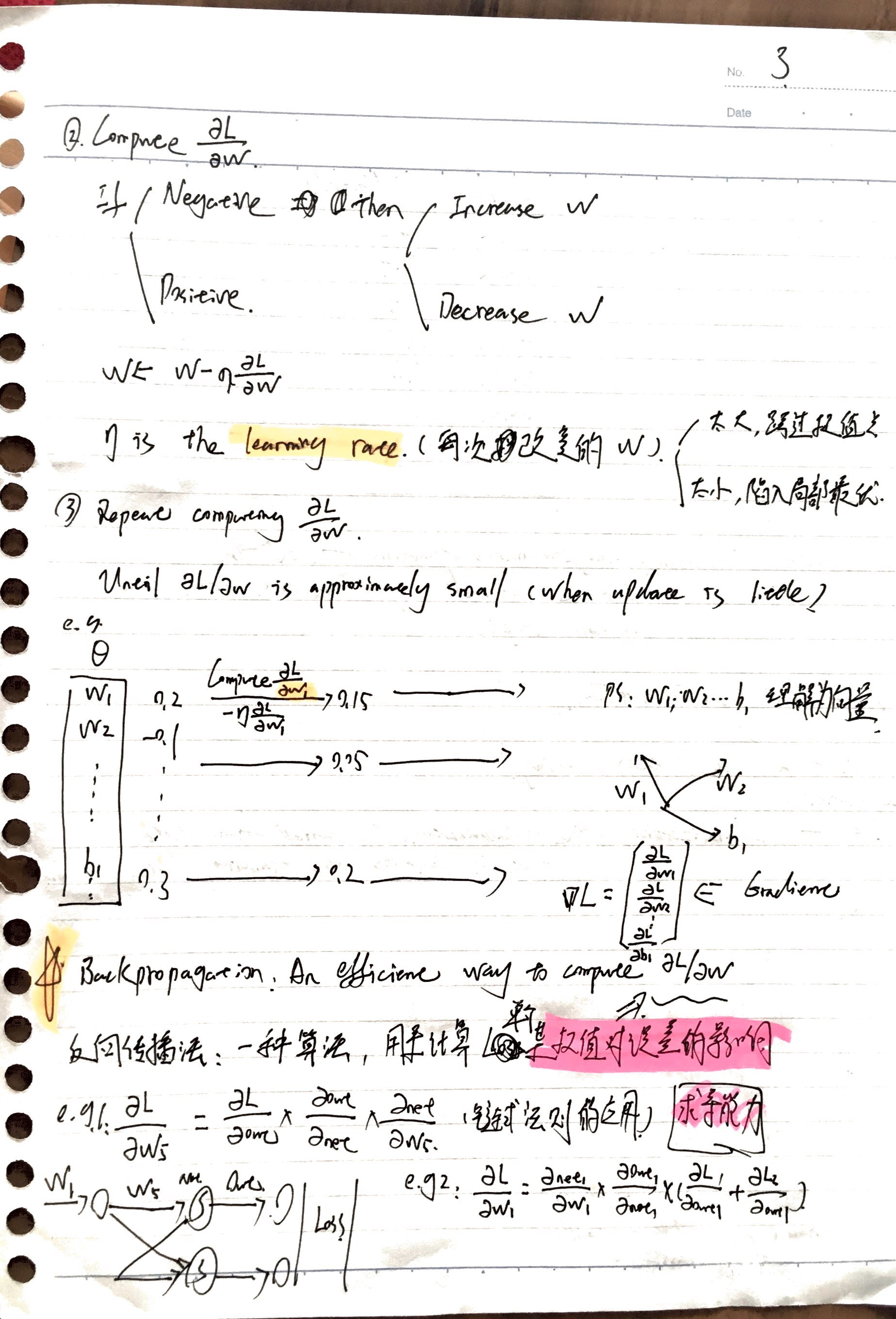展开全文• 本人训练过一些深度学习神经网络的例子，既有单纯全连接网络，又有卷积神经网络加全连接网络，也有通过迁移学习来构建的网络。有时会发散，也就是说有关参数越来越大，产生Nan溢出。表现为激活值（也就是前向网络的...
本人训练过一些深度学习神经网络的例子，既有单纯全连接网络，又有卷积神经网络加全连接网络，也有通过迁移学习来构建的网络。有时会发散，也就是说有关参数越来越大，产生Nan溢出。表现为激活值（也就是前向网络的输出）和代价函数（loss、cost）溢出。基本上可以通过修改超参数来避免。
1 全连接网络的乘法因子矩阵不能初始化为零，要初始化为一个满足正态分布的随机数集，标准差不能太大。训练mnist识别卷积网络时标准差是0.1，训练顺利。使用mnist的训练出来的CNN模型迁移学习识别字母数字混合的字符集时，使用0.1就会造成网络发散，后面改为0.01作为标准差，成功训练。 2 学习率，当你发现loss随着训练过程越来越大，准确率不升高时，最后极有可能会造成激活值溢出，这时你要调低学习率，再训练。同样，mnist训练时的初始学习率0.1可以训练成功，迁移学习网络要设为0.08才不发散。3 使用下面两行的方法计算交叉熵，它可以把ynew 限制在一个不要太小的值和1.0之间。         ynew1 = tf.clip_by_value(ynew,1e-12,1.0)         cross_entropy = -tf.reduce_mean( tf.reduce_sum( y_*tf.log(ynew1) ,1 ) )    而不要使用tf.nn.softmax_cross_entropy_with_logits_v2，这个没说限制在多小的值以上，但是看结果是非常的小。具体可看：  https://blog.csdn.net/brooknew/article/details/82464367
3 推荐使用tf.nn.softmax_cross_entropy_with_logits_v2计算交叉熵。虽然https://blog.csdn.net/brooknew/article/details/82464367的例子表明tf.nn.softmax_cross_entropy_with_logits_v2算出来的loss比较大，但这个例子只有前向传播，没有和后向传播的优化函数结合。在实际训练中，类似tf.train.GradientDescentOptimizer这样的优化器搭配tf.nn.softmax_cross_entropy_with_logits_v2计算loss更不容易发散，可能内含的softMax计算在优化时也会做某种clip的动作。笔者开始使用ynew1 = tf.clip_by_value(ynew,1e-12,1.0)        cross_entropy = -tf.reduce_mean( tf.reduce_sum( y_*tf.log(ynew1) ,1 ) ) 这种方法训练时需要不断的采用1和2的方法调参才不至于发散。
展开全文超参数
• 深度学习神经网络从欠拟合到拟合的调整方法及示例代码 1. 深度神经网络结构调整说明 当深度神经网络可以对少量样本进行很好的拟合，而对较多的样本无法很好拟合的时候，很可能是深度网络的层数不够深，即深度网络...
深度学习神经网络从欠拟合到拟合的调整方法及示例代码
1.     深度神经网络结构调整说明
当深度神经网络可以对少量样本进行很好的拟合，而对较多的样本无法很好拟合的时候，很可能是深度网络的层数不够深，即深度网络的特征无法很好地表征所有样本的特征。此时，可以通过加深网络的层次来使得深度网络对较多的样本进行拟合。
另外，深度网络对于分类样本的拟合能力强于对于连续值样本的拟合能力。即同样的深度网络如果能拟合10组分类的样本，可能只能拟合3组连续值的样本。分类样本采用softmaxwithloss作为损失函数，连续值样本采用欧式距离损失函数。以下例子已连续值样本作为拟合的方式。
2.     少样本合适拟合的示例代码
本例包含四组样本，深度网络较好地拟合。网络参数为：
netsize=[inputsize,5,6,8,7,5,4];
(1)   Main_function
clear all
clc
close all
TrainData =[1   2 3   4   5  6  7   8 9  10
1   9  17 25  33  41  49  57 65  73];
batchsize=4;
TrainData=TrainData(:,1:batchsize);
TrainLabel=[1    0.3  0.8  0.2;
1.8  0.8   1.2 1.1];
classnum=2;%输出端数目

%获取数据的维度
inputsize=size(TrainData ,1);
%获取数据的数量
datanum=size(TrainData ,2);
% 用一个向量来定义网络的深度，以及每层神经元数目。
netsize=[inputsize,5,6,8,7,5,4];
% netsize=[inputsize,5,6,8,9,9,8,7,5,4];

%网络最后一层神经元数数目，再考虑一个偏置。
lastsize=netsize(end)+1;

%初始化网络参数，以结构体的形式保存。
stack = initializeNet(netsize);

% 在训练时，往往需要将参数转成一列向量，提供给损失函数。
% stack ->stackTheta，netconfig保存一些结构参数。
[stackTheta, netconfig] =stack2params(stack);

% 指定固定的最后一层的初始化的值;
rand('state',2)
lastTheta = 0.0005 * randn(lastsize *classnum, 1);

%最终网络需要的参数
Theta=[ lastTheta ; stackTheta ];
%lastTheta表示深度网络最后一层的权值

% the following part is for the traingepoch.
% batchsize=5;
%%每次训练的小批量样本数</span>
batchnum=floor(size(TrainData,2)/batchsize);
DataNum=size(TrainData,2);
alpha=1e-2;
%这是学习率，一般随着网络的悬念都需要不断的减小
lambda = 1e-4; % Weight decay parameter
for epoch=1:16000
v_epoch=epoch
if epoch>=16000
alpha=1e-3;
end
idx=randperm(DataNum);
for t=1:batchnum
subdata=TrainData(:,idx((t-1)*batchsize+1:(t)*batchsize));
sublabel=TrainLabel(:,idx((t-1)*batchsize+1:(t)*batchsize));
end
end
plot(log(cost))

(2)   drelu
function dre= drelu(x)
dre=zeros(size(x));
dre(x>0)=1;
dre(x==0)=0.5; %这句可以不要
end
(3)   relu
function re = relu(x)
re = max(x,0)-1;
end
(4)   params2stack
function stack = params2stack(params, netconfig)
depth =numel(netconfig.layersizes);
stack = cell(depth,1);
prevLayerSize =netconfig.inputsize;  %the size of the previous layer
curPos = double(1); % mark current position in parametervector
for d = 1:depth
% Create layer d
stack{d} = struct;
% Extract weights
wlen =double(netconfig.layersizes{d} * prevLayerSize);
stack{d}.w =reshape(params(curPos:curPos+wlen-1), netconfig.layersizes{d}, prevLayerSize);
curPos = curPos+wlen;
% Extract bias
blen =double(netconfig.layersizes{d});
stack{d}.b =reshape(params(curPos:curPos+blen-1), netconfig.layersizes{d}, 1);
curPos = curPos+blen;
% Set previous layer size
prevLayerSize =netconfig.layersizes{d};
end
end
(5)   ReLUDNNCost
%参数获取的一些操作
lastTheta =reshape(theta(1:lasthiddenSize*numClasses), numClasses, lasthiddenSize);
%从theta向量中抽取网络权值参数并转化
stack =params2stack(theta(lasthiddenSize*numClasses+1:end), netconfig);

%这里保存在应用BP算法求梯度时需要的数据
PARA=cell(numel(stack),1);
%传进来的样本数
datanum=size(trainData,2);

%开始前馈，网络虽然多层，但只是重复而已
data=trainData;
for d = 1:numel(stack)
PARA{d}.a=data;
z2=(stack{d}.w*data)+stack{d}.b*ones(1,datanum);
%ReLU函数
a2=relu(z2);
data=a2;
%ReLU函数的导函数
PARA{d}.daz=drelu(z2);
end
a2=[a2;ones(1,datanum)];
%开始求解损失
groundTruth=trainLabels;
v_groundTruth=groundTruth
M = lastTheta*a2;
v_M=M

% 损失函数，
cost=sum(sum((groundTruth-M).^2))./datanum;
%最后一层神经元的目标函数对lastTheta 的导数，
% 输出层误差传导至倒数第二层神经元的值
predelta=-lastTheta'*(groundTruth-M);

predelta=predelta(1:end-1,:);
for d = numel(stack):-1:1
delta=predelta.*PARA{d}.daz;
predelta=stack{d}.w'*delta;
end
end
(6)   stack2params
function [params, netconfig] = stack2params(stack)
params = [];
for d = 1:numel(stack)
params = [params ; stack{d}.w(:) ;
stack{d}.b(:) ];
end
if nargout > 1
if numel(stack) == 0
netconfig.inputsize = 0;
netconfig.layersizes = {};
else
netconfig.inputsize = size(stack{1}.w,2);
netconfig.layersizes = {};
for d =1:numel(stack)
netconfig.layersizes =[netconfig.layersizes ; size(stack{d}.w,1)];
end
end
end
end

(7)   initializeNet
function stack = initializeNet(netsize)
layersize=length(netsize(:));
stack = cell(layersize-1,1);
for l=1:layersize-1
hiddenSize=netsize(l+1);
visibleSize=netsize(l);
r =sqrt(6) /sqrt(hiddenSize+visibleSize+1);
rand('state',2)
stack{l}.w= rand(hiddenSize, visibleSize) *2 * r - r; stack{l}.b= zeros(hiddenSize, 1);
end
end

(8)   运行结果
深度网络收敛，运行结果如下图所示：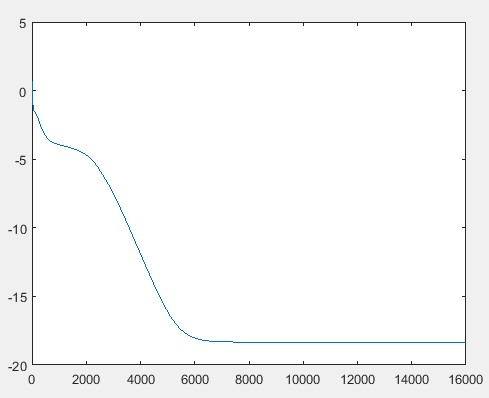(F1)
输出标签值如下：
v_groundTruth =
0.2000    0.3000    1.0000   0.8000
1.1000    0.8000    1.8000   1.2000
深度网络实际拟合值如下：
v_M =
0.2001    0.3001    0.9999   0.7999
1.0999    0.8000   1.7999    1.2000
由上面数据比较可见，两者差距较小，深度网络较好地拟合。

3.     多样本深度网络欠拟合代码
本例在第2部分中的四组样本的基础上，增加一组样本，深度网络无法拟合。网络参数为：
netsize=[inputsize,5,6,8,7,5,4];
(1)   Main_function
clear all
clc
close all
TrainData=[1   2 3   4   5  6  7   8 9  10
1  9  17  25 33  41 49  57 65 73];
batchsize=5;
TrainData=TrainData(:,1:batchsize);
TrainLabel=[1    0.3  0.8  0.2  0.67;
1.8 0.8   1.2  1.1 0.18];
classnum=2;%输出端数目

%获取数据的维度
inputsize=size(TrainData,1);
%获取数据的数量
datanum=size(TrainData,2);
% 用一个向量来定义网络的深度，以及每层神经元数目。
netsize=[inputsize,5,6,8,7,5,4];
% netsize=[inputsize,5,6,8,9,9,8,7,5,4];

%网络最后一层神经元数数目，再考虑一个偏置。
lastsize=netsize(end)+1;

%初始化网络参数，以结构体的形式保存。
stack =initializeNet(netsize);

% 在训练时，往往需要将参数转成一列向量，提供给损失函数。
% stack->stackTheta，netconfig保存一些结构参数。
[stackTheta,netconfig] = stack2params(stack);

% 指定固定的最后一层的初始化的值;
rand('state',2)
lastTheta =0.0005 * randn(lastsize * classnum, 1);

%最终网络需要的参数
Theta=[lastTheta ; stackTheta ];
%lastTheta表示深度网络最后一层的权值

% the followingpart is for the traing epoch.
% batchsize=5;
%%每次训练的小批量样本数</span>
batchnum=floor(size(TrainData,2)/batchsize);
DataNum=size(TrainData,2);
alpha=1e-2;
%这是学习率，一般随着网络的悬念都需要不断的减小
lambda = 1e-4; %Weight decay parameter
forepoch=1:16000
v_epoch=epoch
if epoch>=16000
alpha=1e-3;
end
idx=randperm(DataNum);
for t=1:batchnum
subdata=TrainData(:,idx((t-1)*batchsize+1:(t)*batchsize));
sublabel=TrainLabel(:,idx((t-1)*batchsize+1:(t)*batchsize));
end
end
plot(log(cost))

(2)   运行结果
深度网络无法很好地收敛，运行结果如下图所示：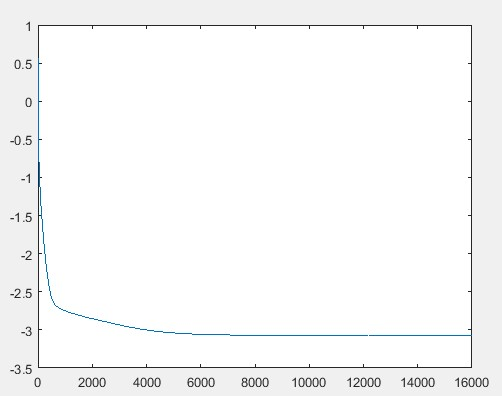(F2)
输出标签值如下：
v_groundTruth =
0.6700   0.3000    0.2000    1.0000   0.8000
0.1800    0.8000   1.1000    1.8000    1.2000
深度网络实际输出值如下
v_M =
0.6114    0.4340    0.4819   0.9996    0.4429
0.1749    0.8124   1.1275    1.8001    1.1651
由上面数据比较可见，两者差距较大，深度网络无法很好地拟合。
4.     加深网络后多样本可以很好地拟合
本例在第3部分中的五组样本，深度网络无法拟合的情况下，加深网络深度，网络参数为：
netsize=[inputsize,5,6,8,9,9,8,7,5,4]; 深度网络很好地拟合。

(1)   Main_function
clear all
clc
close all
TrainData =[1   2 3   4   5  6  7   8 9  10
1   9  17 25  33  41 49 57 65 73];
% TrainData=TrainData(:,1:6);
% TrainLabel=[1 0.3 1.8 0.87 0.66 0.83];
% batchsize=6;
% classnum=1;%输出端数目
batchsize=5;
TrainData=TrainData(:,1:batchsize);
TrainLabel=[1    0.3  0.8  0.2  0.67;
1.8  0.8   1.2 1.1  0.18];
% TrainLabel=[1    0.3  0.8  0.2;
%             1.8  0.8  1.2  1.1];
classnum=2;%输出端数目

%获取数据的维度
inputsize=size(TrainData ,1);
%获取数据的数量
datanum=size(TrainData ,2);
% 用一个向量来定义网络的深度，以及每层神经元数目。
netsize=[inputsize,5,6,8,9,9,8,7,5,4];

%网络最后一层神经元数数目，再考虑一个偏置。
lastsize=netsize(end)+1;

%初始化网络参数，以结构体的形式保存。
stack = initializeNet(netsize);

% 在训练时，往往需要将参数转成一列向量，提供给损失函数。
% stack ->stackTheta，netconfig保存一些结构参数。
[stackTheta, netconfig] =stack2params(stack);

% 指定固定的最后一层的初始化的值;
rand('state',2)
lastTheta = 0.0005 * randn(lastsize *classnum, 1);

%最终网络需要的参数
Theta=[ lastTheta ; stackTheta ];
%lastTheta表示深度网络最后一层的权值

% the following part is for the traing epoch.
% batchsize=5;
%%每次训练的小批量样本数</span>
batchnum=floor(size(TrainData,2)/batchsize);
DataNum=size(TrainData,2);
alpha=1e-2;
%这是学习率，一般随着网络的悬念都需要不断的减小
lambda = 1e-4; % Weight decay parameter
for epoch=1:16000
v_epoch=epoch
if epoch>=16000
alpha=1e-3;
end
idx=randperm(DataNum);
for t=1:batchnum
subdata=TrainData(:,idx((t-1)*batchsize+1:(t)*batchsize));
sublabel=TrainLabel(:,idx((t-1)*batchsize+1:(t)*batchsize));
end
end
plot(log(cost))

(2)   运行结果
深度网络很好地收敛，运行结果如下图所示：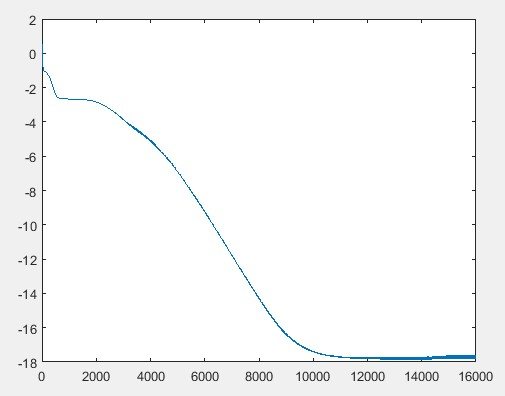(F3)
输出标签值如下：
v_groundTruth =
0.6700    0.3000    0.2000   1.0000    0.8000
0.1800    0.8000   1.1000    1.8000    1.2000
深度网络实际输出值如下：
v_M =
0.6699    0.3000   0.2002    0.9999    0.7999
0.1800    0.8000   1.0999    1.7998    1.2001
由上面数据比较可见，两者差距较小，深度网络很好地拟合。
5.     总结
从第2部分至第5部分的训练样本及运行结果可以看出，当深度网络较浅时，可能会面临多样本数据无法很好拟合的情况。此时，如果加深网络层数，则可能会使得网络很好地拟合多组训练样本。
展开全文matlab
• 主要问题DNN（Deep Neural Networks）的冗余性决定了参数个数不会对网络的精度造成影响。此外，SGD（Stochastic Gradient Descent）所需要的精度仅为6~8bit，因此合理的量化网络也可保证精度的情况下减小模型的存储...
主要问题
影响
DNN压缩看参考Stanford大学Han Song博士的几篇论文，如下：
Learning both Weights and Connections for Efficient Neural NetworksRegularizing Deep Neural Networks with Dense-Sparse-Dense TrainingCompressing Deep Neural Networks with Pruning , Trained Quantization and Huffman Coding
算法的主要实现思路是发现对网络贡献较小的参数并精简，然后重新训练精简后的稀疏网络以达到减少非0网络参数的个数并保留或提高网络精度的目的，此类方法属于改变网络密度类方法。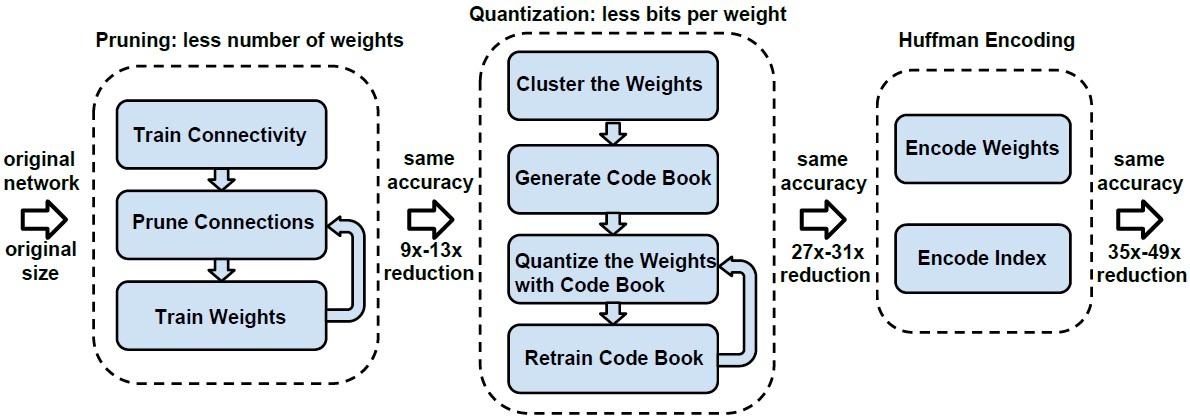图1 Deep Compression的深度神经网络剪枝，量化和编码的示意框图。
量化技术
深度神经网络的量化技术主要可以分为两类：完整训练后量化和训练时量化。与改变密度类方法不同，量化类的方法属于改变网络多样性的方法，容易造成精度损失。  完整训练神经网络后，再执行量化类技术主要针对已完整训练的网络
https://www.zhihu.com/question/40503595
展开全文• 1）图像识别用途甚广，解决的算法之一，是深度学习神经网络。matlab近几个版本，对这块的语法修改较多，总体而言，用户用起来更方便了； 2）这里以2018a版本为例，做一些粗略的说明。 1.概念说明 1）图像识别：图像...
• 测试程序也是很简单。 首先创建计算图： ...with tf.Graph().as_default() as g: ...计算图的输入和输出占位与之前反向传播的函数一样： ... y_ = tf.placeholder(tf....下面是关于更有挑战性的卷积神经网络的构建。
• if __name__ == "__main__": parameters = model(train_X, train_Y, initialization="random") print ("On the train set:") predictions_train = predict(train_X, train_Y, parameters) print ("On the test ...python
• 你的反向传播效果非常好! difference = 1.18904178766e-07shell
• 现如今，人工智能/深度学习/Deep Learning 异常火爆，可惜是网络上绝大部分推荐的入门书籍/课程都是英文的，本来数学基础就不行，又是英文资料着实让人头疼。这里咪博士向大家推荐 2 份非常不错的中文入门资料。是的...人工智能 机器学习
• 本文我们聊聊如何才能画出炫酷高大上的神经网络图，下面是常用的几种工具。 这个工具可以非常方便的画出各种类型的图，是下面这位小哥哥开发的，来自于麻省理工学院弗兰克尔生物工程实验室, 该实验室开发可视化和...
• 神经网络深度学习 神经网络：一种可以通过观测数据使计算机学习的仿生语言范例 深度学习：一组强大的神经网络学习技术神经网络深度学习目前提供了针对图像识别，语音识别和自然语言处理领域诸多问题的最佳解决...自然语言处理
• 是一种常见的深度学习架构，受生物自然视觉认知机制(动物视觉皮层细胞负责检测光学信号)启发而来，是一种特殊的多层前馈神经网络。它的人工神经元可以响应一部分覆盖范围内的周围单元，对于大型图像处理有出色表现...图像处理 网络
• 深度学习神经网络，多层神经网络，BP,卷积神经网络区别接触神经网络不久，想弄明白“深度学习”与”多层神经网络”的区别，看了几篇博客和知乎回答，总结一下自己的认识 BP是指多层前馈神经网络神经网络包括了多层...
• 吴恩达神经网络深度学习——浅层神经网络深层神经网络 深层神经网络
• 深度学习（DL）：深度学习是机器学习的一个分支，是指一类问题以及解决这类问题的方法。 神经网络（ANNs）：又称人工神经网络，是由人工神经元以及神经元之间的连接构成,其中有两类特殊的神经元: 一类是用来接受外部...人工智能 机器学习
• 掌握使用keras解决深度学习主要问题（神经网络、卷积神经网络、循环神经网络），以及深度学习主要内容：神经网络、卷积神经网络、循环神经网络；案例简介。   第二部分，深度学习之多层感知器(MLP)。主要学习...RNN 人工智能
• ## 神经网络和深度学习基本原理

万次阅读 多人点赞 2018-11-12 16:58:19
这是看到的一篇对神经网络的讲解的文章，我觉得写得很好，也仔细学习了... 神经网络深度学习目前提供了针对图像识别，语音识别和自然语言处理领域诸多问题的最佳解决方案。传统的编程方法中，我们告诉计算机如何...
• AlexNet网络现代意义上的深度卷积神经网络起源于AlexNet网络，它是深度卷积神经网络的鼻祖。这个网络相比之前的卷积网络最显著的特点是层次加深，参数规模变大。网络结构如下图所示：这个网络有5个卷积层，它们中的...
• 激活函数神经网络神经元中，输入的 inputs 通过加权，求和后，还被作用了一...假设一个示例神经网络中仅包含线性卷积和全连接运算，那么该网络仅能够表达线性映射，即便增加网络的深度也依旧还是线性映射，难以有效建模激活函数
• 一、人工智能、机器学习和深度学习 人工智能是很宽泛的领域，让机器像人一样思考：机器学习，自动推理；让机器人像人一样听懂：...深度学习属于机器学习的子类，是利用深度神经网络来解决特征表达的一种学习过程。...机器学习 人工智能
• 最近在学习复旦邱锡鹏老师的《神经网络深度学习》，并对书中推荐的论文进行了阅读。感觉这本书对深度学习讲解得很好，即有全面严谨的讲述，也有高屋建瓴的理解。 本文的行文结构是按邱老师的书籍结构来的，并...
• 从广义上说深度学习的网络结构也是多层神经网络的一种。 传统意义上的多层神经网络是只有输入层、隐藏层、输出层。其中隐藏层的层数根据需要而定，没有明确的理论推导来说明到底多少层合适。 而深度学习中最著名的...
•python
• 一般有前馈神经网络FFNN模型（feedforward neural network），隐层多的可以叫深度神经网络DNN（deep nn）。 固定基函数的线性组合构成的回归模型和分类模型。我们看到,这些模型具有一些有用的分析性质和计算性质,...DNN
• 神经网络深度学习区别： 这两个概念实际上是互相交叉的，例如，卷积神经网络（Convolutional neural networks，简称CNNs）就是一种深度的监督学习下的机器学习模型，而深度置信网（Deep Belief Nets，简称DBNs）...机器学习
• ## 深度学习与围棋：神经网络入门

千次阅读 多人点赞 2021-03-02 07:42:18
这类算法对现代深度学习至关重要。人工神经网络的历史可以追溯到20世纪40年代早期。历经数十年，它在许多领域的应用都取得了巨大成功，但其基本思想都保留了下来。 人工神经网络的核心理念，是从神经科学中汲取灵感...alphago 人工智能
• 文章目录一、前言二、LSTM的是什么三、准备工作1.设置GPU2.设置相关参数3.加载数据四、数据预处理1.归一化2.时间戳函数五、构建模型六、激活模型七、训练模型八、结果可视化1....来自专栏：【深度学习LSTM lstm 1024程序员节...